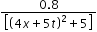Courses

# Test: Behavior Of Progressive Waves

## 10 Questions MCQ Test Physics For JEE | Test: Behavior Of Progressive Waves

Description
This mock test of Test: Behavior Of Progressive Waves for JEE helps you for every JEE entrance exam. This contains 10 Multiple Choice Questions for JEE Test: Behavior Of Progressive Waves (mcq) to study with solutions a complete question bank. The solved questions answers in this Test: Behavior Of Progressive Waves quiz give you a good mix of easy questions and tough questions. JEE students definitely take this Test: Behavior Of Progressive Waves exercise for a better result in the exam. You can find other Test: Behavior Of Progressive Waves extra questions, long questions & short questions for JEE on EduRev as well by searching above.
QUESTION: 1

Solution:
QUESTION: 2

### The disc of a siren containing 60 holes rotates at a constant speed of 360 rpm. The emitted sound is in unison with a tuning fork of frequency.

Solution:

Frequency of revolution of disc = 360 rpm = 360 / 60rps = 60rps
Frequency of emitted sound = 6 × No.of holes
= 6 ×60 = 360Hz.

QUESTION: 3

### The speed of transverse waves in a string depends on:

Solution:

For a string v = √(T/μ)

QUESTION: 4

Speed of the sound in a perfectly rigid rod would be

Solution:

Since particles in a rigid body do not move and hence, no time is delayed in travelling of sound waves.

QUESTION: 5

Which instrument work on the basis of reflection of sound waves?

Solution:

Stethoscope works on the basis of reflection of sound waves.

QUESTION: 6

Speed of a progressive wave,v is given by

Solution:

f = v/λ
v = λf

QUESTION: 7

What causes the rolling sound of thunder?

Solution:

The air is not of uniform density as we go upwards. as we go upwards there is a gradual decrease in air density which results in refraction thereby leading to the rolling sound of thunder.

QUESTION: 8

Properties of a kind of wave are given below. Identify the type of wave

i. Wave can not be detected by human ear
ii. Velocity of wave in free space is 3x108m/s
iii. Velocity is not effected by temperature
iv. Waves can travel through vacuum

Solution:

These are the properties of radio waves. No possible explanation.

QUESTION: 9

Y (x,t) =Syntax error from line 1 column 49 to line 1 column 73. Unexpected ‘mathsize’. represents a moving pulse where X and y are n metres and t in second. Then which of the following dose not hold true?

Solution:

t = 0 & x = 0
y = 0.16 m
ymax = 0.16 m
Pulse is symmetric because y (x) = y (-x)
t = 1s
x=-1.25m
y is again 0.16m
Therefore speed is 1.25m/s
So it travels 2.5 m in 2 sec

QUESTION: 10

The speed of transverse waves on a string depends upon

Solution:

The speed of a wave on a string depends on the square root of the tension divided by the mass per length, the linear density.